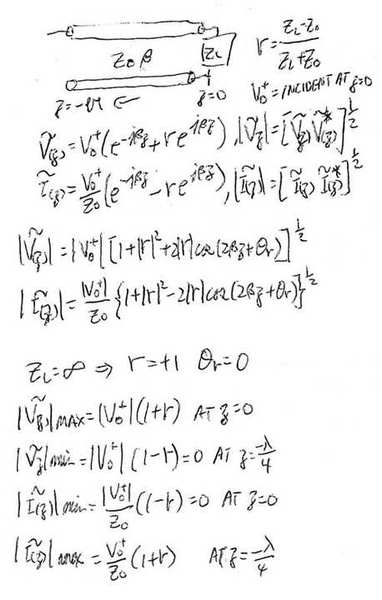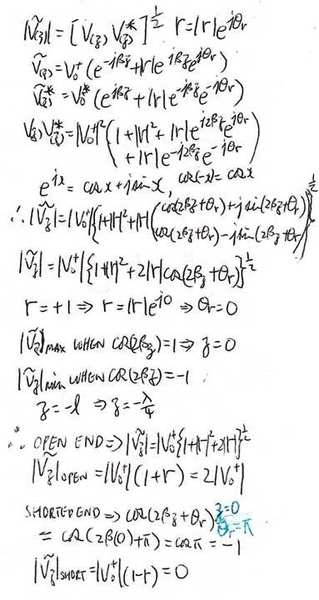# Waves in a coaxial cable: Boundary conditions

## Homework Statement

Hi all.

I have the following expression, which relates the incoming amplitude with the reflected amplitude at a point x = L in a coaxial cable:

$$A_{\text{reflected}} = \frac{R-Z_0}{R+Z_0}A_{\text{incoming}}.$$

Here R is the resistance at the point x = L and Z0 is the characteristic impedance of the cable.

Lets say that I do not terminate the cable at point x = L, i.e. it is just left open. Then R = infinity, which gives me that $A_{\text{reflected}}=A_{\text{incoming}}$.

I know that the current I is zero at the point x=L (since the cable is left open, no current can run thorugh here), but how does this match up with the fact that $A_{\text{reflected}}=A_{\text{incoming}}$?

Best regards,
Niles.

## Homework Statement

Hi all.

I have the following expression, which relates the incoming amplitude with the reflected amplitude at a point x = L in a coaxial cable:

$$A_{\text{reflected}} = \frac{R-Z_0}{R+Z_0}A_{\text{incoming}}.$$

Here R is the resistance at the point x = L and Z0 is the characteristic impedance of the cable.

Lets say that I do not terminate the cable at point x = L, i.e. it is just left open. Then R = infinity, which gives me that $A_{\text{reflected}}=A_{\text{incoming}}$.

I know that the current I is zero at the point x=L (since the cable is left open, no current can run thorugh here), but how does this match up with the fact that $A_{\text{reflected}}=A_{\text{incoming}}$?

Best regards,
Niles.

This is really a good one. I am studying EM day and night for the last 8 months. Just take for granted this is the truth!!!

Well look at it this way: Think of it as power wave ( you will encounter later on by taking voltage and Z0). When you sent power out and all of a sudden the road ended!!! Power is conservative, it has to go somewhere, so it got reflected back the original path.....road....coax!!! Remember, when you are dealing with microwave, it does take time to travel through coax ( about 8 in/nsec depend of material). When you launch a wave, you cannot take it back, the wave has to run through the whole path....from the launch end, through the coax, encounter the open, reflected back. So the reflected wave sum with the wave that is still travelling forward and create a standing wave pattern.

I am not sure whether I even explained correctly. I am still learning. I don't think it would help you if I dump more equation on you. So this is the over simplified approach!!!

First of all, I forgot to mention that A is the amplitude of the voltage.

And second, my issue is that I can't see how a total reflection (without a phase change) of the voltage can make the current be zero in that point.

First of all, I forgot to mention that A is the amplitude of the voltage.

And second, my issue is that I can't see how a total reflection (without a phase change) of the voltage can make the current be zero in that point.

You did wrote A-incoming.

Current and voltage on the line are not in phase. If you look at the travelling voltage and current wave equation, there is a sign difference in the second term.

I think you better write out the equation to show where your question is so I can make sure I understand your question. Look at the standing wave pattern for open termination and see whether that help.

Ok, the amplitude of the reflected is related to the incoming voltage by:

$$V_{\text{reflected}} = \frac{R-Z_0}{R+Z_0}V_{\text{incoming}}.$$

Thus when the cable just ends (no termination), we get that $V_{\text{reflected}}=V_{\text{incoming}}$. So all voltage gets reflected without a phase change. How does one conclude from this that the current in the point where the voltage is reflected (i.e. at the point x = L, where the cable is ended) must be zero?

Ok, the amplitude of the reflected is related to the incoming voltage by:

$$V_{\text{reflected}} = \frac{R-Z_0}{R+Z_0}V_{\text{incoming}}.$$

Thus when the cable just ends (no termination), we get that $V_{\text{reflected}}=V_{\text{incoming}}$. So all voltage gets reflected without a phase change. How does one conclude from this that the current in the point where the voltage is reflected (i.e. at the point x = L, where the cable is ended) must be zero?

For this I have no choice but to go into travelling wave equation to get the amplitudes of voltage and current.As you can see, the voltage max at the load end at z=0 and correspond to current equal 0. You need to think of the travelling wave is travelling with finite speed. The current has a different phase than the voltage because the sign on the second term is -ve. Hope that help.

read up the travelling wave and standing wave ratio in RF books, I have not seen any books that successfully described this in English yet. You just have to work with the equation to get the feel.

If anyone can give a good intuitive description, I would like to read it too.

This kind of topic, you have to go over and over and over again to try to get the feel of it. I myself bought six or seven books and read through the same topic, work through the homeworks over and over to get the feel and I still cannot say I understand totally.

I know a friend in the gym, he has a PhD in Physics and he is the director of a big project involve EM and radar. He told me when he was studying electrodynamics with the book by JD Jackson, he had to read it 15 times. When he was a TA, students asked him what to do to understand this, he said read 15 times!!!! I spent the money buying all different books and read them, each have different approach, so hopefully after reading it and write out their equations, you would get a feel.

Last edited:
Thanks for taking the time to write all this down. Is there any way I can make you upload a larger image?

Thanks again, I really appreciate it.

Thanks for taking the time to write all this down. Is there any way I can make you upload a larger image?

Thanks again, I really appreciate it.

I updated the picture. Is this better. I want to make sure this is what you are looking for. If you have any more question on this, I am more than happy to elaborate the equations much further. I did skip a lot of in between steps to give you a quick and fast answer so far.

Is it exactly what I am asking for, but there are some steps in your derivation that I do not get; it is the part where you find |V| and |I|, and how you deduce |V|_max.

By the way, I will log of in not so long time (I must get some sleep), but I'll look here first thing tomorrow. Thanks so far.

Is it exactly what I am asking for, but there are some steps in your derivation that I do not get; it is the part where you find |V| and |I|, and how you deduce |V|_max.

By the way, I will log of in not so long time (I must get some sleep), but I'll look here first thing tomorrow. Thanks so far.Last edited:
Thanks! I understand it now; I really appreciate it!

By the way yungman, I have one more question, and it is related to this topic.

When the cable just ends (i.e. no termination), we found that the current in the point must be zero, since the resistance is infinite. But what about the potential?

And when the cable is short circuited (i.e. the resistance is zero), then the potential is zero. What about the current in this case?

Ahh, I should just look at your derivation.. at the point where the cable ends, the voltage is maximum.

It is a nice method you've taught me. Thanks again.

Ahh, I should just look at your derivation.. at the point where the cable ends, the voltage is maximum.

It is a nice method you've taught me. Thanks again.

I update the original equations again. Read it over and see what you think.

Where are you? You go to sleep when I am wild awake!!

It's great; I got the same results as you, but that's because I used your method.

I'm in Scandinavia (northern Europe), so there's still ~2 hours more before I have to sleepIt's great; I got the same results as you, but that's because I used your method.

I'm in Scandinavia (northern Europe), so there's still ~2 hours more before I have to sleepWelcome here from the other side of the world!!

Always verify my stuff, I am learning too. I have not seen any books derive these yet. I spent some time doing it and keep checking with 5 or 6 books already, but that does not mean I am 100% correct. I just cannot find any book that work out the equations step by step yet. that is the part that takes the most time. I just cannot take the books in face value without working out the equation.

If you are interested in these stuff, I'll likely to post a question in the Electrical Engineer section. I think that is more suitable for this kind of EM stuff. I have something that I have been working on and I still don't quite agree with the book yet. It is similar to this stuff here. I am going to spent a little more time and I am going to post it there. Feel free to join in.

The three main books that I use are 1) Electromagnetics for Engineer by Fawwaz Ulaby and 2) Field and Waves Electromagnetics by David K Cheng. 3) A very good RF book is Microwave Engineering by David Pozar. These have the most formulas .

I reference a lot on Introductory Electromagnetics by Zoya Popovic and Electromagnetics for Engineers by Steven Schwarz.

Last edited:
Thanks for the references. I'm using Griffiths Introduction to Electrodynamics, and although it is well written, the book isn't dedicated to EM-waves (it discusses eletrostatics as well).

Thanks for the references. I'm using Griffiths Introduction to Electrodynamics, and although it is well written, the book isn't dedicated to EM-waves (it discusses eletrostatics as well).

I got that one also, I got it only a month ago and have not use it a lot yet. Seems like a good book, easier than JD Jackson.

This kind of phasor stuff is more common in microwave electronics. The transmission line theory is very into voltage and current phasor. They are very similar to EM phasor. Them even do a comparison between the two. There you get more information. All the formulas are very similar. Pozar is one of those. Actually it has a chapter on EM also. I am into microwave electronics not that much of a physics guy!!!